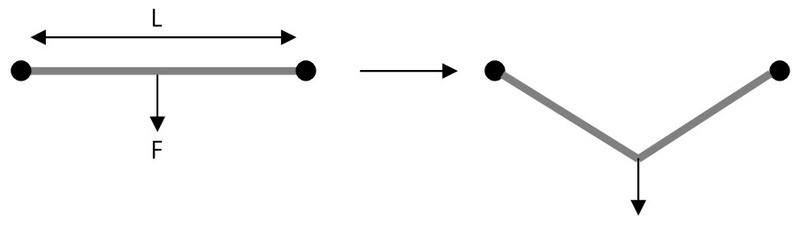# Horizontal cable with one vertical point force

## Homework Statement

Hang a weight (F) from the middle of a horizontal wire of length L, attached on both sides. The wire has an effective modulus (E) and effective area (A). Find an equation for the new length of the wire LF. (NOTE: Do not try to solve for a form LF =, this is too hard).## Homework Equations

To see the equations I thought were relevant, see my attempt at the solution.

## The Attempt at a Solution

Force Balance Equation: T = $\frac{Fcosθ}{2}$

Compatibility Equation: ∂ = ∂1 + ∂2. ∂1 = ∂2.

Force-Displacement Equation: ∂1 = $\frac{TL}{2EA}$ = $\frac{FLcosθ}{4EA}$.

This gives: ∂=2∂1 = $\frac{FLcosθ}{2EA}$

Which gives a final answer of: LF=L+∂ = L + $\frac{FLcosθ}{2EA}$.

But I'm not confident in my work, because professor said it would be too difficult to solve for the solution in this form.

Spinnor
Gold Member
From where do you measure θ? What is θ when F goes to zero?

Theta is the angle made at the support ends. Theta is the same on the left and right side. When F=0, Theta=0, theoretically.

Spinnor
Gold Member
Shouldn't you have then,

2Tsinθ = F

Okay I've got the same problem as part of a homework.
I agree that you need 2Tsin(A)=F

So then, with the new force balance equation, we get:

1 = $\frac{TL}{2EA}$ = $\frac{FL}{4EAsinθ}$

Which gives us:

∂=∂1 + ∂2 = 2∂1 = $\frac{FL}{2EAsinθ}$

So the final answer then would be:

LF=L+$\frac{LF}{2EAsinθ}$